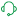# New to QlikView

Discussion board where members can get started with QlikView.

Announcements
Modernize Your QlikView Deployment webinar, Nov. 3rd. REGISTER
cancel
Showing results for
Did you mean:
HighlightedContributor

## different result when a specific date is selected in pivot table

i am calculating market share for all dates of current month but if a specific date is selected the table gives different result for the same date. as you can see in screenshot if I selected a specific Company and a date '02/10/2020' it' giving market share 0.56 in above table and in lower table it's 13.35 which is the correct market share for the date. I want to show correct figures for all the dates of the month in above table. I am not getting what is wrong because formula in both tables is same. I am attaching qvw file for reference.6 Replies
HighlightedContributor

@Kush @sunny_talwar Please have a look

HighlightedMVP

HighlightedContributor

Can you please explain what did you do in the attached solution except total<AppDate> in upper table?

HighlightedContributor

@Kush it didn't solve my problem.

Also I have to calculate cumulative sum

Simple sum Expression is working  but when I apply rangesum formula to caculate Cumulative it's returning 0.

Sum Expression:

SUM(TOTAL<[Invoice Date_FKDAT]>aGGR(((Sum({<RecType={'Sales'},[Posting Status_RFBSK]={'C'},[FISCAL YEAR] = {\$(=Max([FISCAL YEAR]))},[MonthNo] = {\$(=Match(Month(Date(Max([Invoice Date_FKDAT]))), \$(=chr(39) & Replace(MonthNames, ';', chr(39) & ',' & chr(39)) & chr(39))))}
>}[SalesMTon])))
-
((((Sum({<RecType={'Sales'},[Posting Status_RFBSK]={'C'},[FISCAL YEAR] = {\$(=Max([FISCAL YEAR]))},[MonthNo] = {\$(=Match(Month(Date(Max([Invoice Date_FKDAT]))), \$(=chr(39) & Replace(MonthNames, ';', chr(39) & ',' & chr(39)) & chr(39))))},
[Billing Type_FKART]={'S1','RE','ZRAX','ZREX','ZRFX','ZRFI','G2','ZRCO'}>}[SalesMTon]))))*2),[Invoice Date_FKDAT]))

Cumulative sum Expression:

=Rangesum(above(total
(fabs(Sum({<RecType={'Sales'},[Posting Status_RFBSK]={'C'},[FISCAL YEAR] = {\$(=Max([FISCAL YEAR]))},[MonthNo] = {\$(=Match(Month(Date(Max([Invoice Date_FKDAT]))), \$(=chr(39) & Replace(MonthNames, ';', chr(39) & ',' & chr(39)) & chr(39))))}>}[SalesMTon])))
-
(((fabs(Sum({<RecType={'Sales'},[Posting Status_RFBSK]={'C'},[FISCAL YEAR] = {\$(=Max([FISCAL YEAR]))},[MonthNo] = {\$(=Match(Month(Date(Max([Invoice Date_FKDAT]))), \$(=chr(39) & Replace(MonthNames, ';', chr(39) & ',' & chr(39)) & chr(39))))},[Billing Type_FKART]={'S1','RE','ZRAX','ZREX','ZRFX','ZRFI','G2','ZRCO'}>}[SalesMTon]))))*2),0,RowNo(total)))

HighlightedContributor

I have updated my qlikview document. I have two measures in the same expression.

IF(Company='ABC',
((((Sum({<RecType={'Sales'},[Posting Status_RFBSK]={'C'},[FISCAL YEAR] = {\$(=Max([FISCAL YEAR]))},[MonthNo] = {\$(=Match(Month(Date(Max([Invoice Date_FKDAT]))), \$(=chr(39) & Replace(MonthNames, ';', chr(39) & ',' & chr(39)) & chr(39))))}
,[Invoice Date_FKDAT]={">=\$(=Date(vMinDates,'DD/MM/YYYY'))<=\$(=Date(vMaxDates,'DD/MM/YYYY'))"}>}[SalesMTon])))
-
((((Sum({<RecType={'Sales'},[Posting Status_RFBSK]={'C'},[FISCAL YEAR] = {\$(=Max([FISCAL YEAR]))},[MonthNo] = {\$(=Match(Month(Date(Max([Invoice Date_FKDAT]))), \$(=chr(39) & Replace(MonthNames, ';', chr(39) & ',' & chr(39)) & chr(39))))},
[Invoice Date_FKDAT]={">=\$(=Date(vMinDates,'DD/MM/YYYY'))<=\$(=Date(vMaxDates,'DD/MM/YYYY'))"},[Billing Type_FKART]={'S1','RE','ZRAX','ZREX','ZRFX','ZRFI','G2','ZRCO'}>}[SalesMTon]))))*2)))

/
(((((Sum({<RecType={'Sales'},[Posting Status_RFBSK]={'C'},[FISCAL YEAR] = {\$(=Max([FISCAL YEAR]))},[MonthNo] = {\$(=Match(Month(Date(Max([Invoice Date_FKDAT]))), \$(=chr(39) & Replace(MonthNames, ';', chr(39) & ',' & chr(39)) & chr(39))))}
,[Invoice Date_FKDAT]={">=\$(=Date(vMinDates,'DD/MM/YYYY'))<=\$(=Date(vMaxDates,'DD/MM/YYYY'))"}>}[SalesMTon])))
-
((((Sum({<RecType={'Sales'},[Posting Status_RFBSK]={'C'},[FISCAL YEAR] = {\$(=Max([FISCAL YEAR]))},[MonthNo] = {\$(=Match(Month(Date(Max([Invoice Date_FKDAT]))), \$(=chr(39) & Replace(MonthNames, ';', chr(39) & ',' & chr(39)) & chr(39))))},
[Invoice Date_FKDAT]={">=\$(=Date(vMinDates,'DD/MM/YYYY'))<=\$(=Date(vMaxDates,'DD/MM/YYYY'))"},[Billing Type_FKART]={'S1','RE','ZRAX','ZREX','ZRFX','ZRFI','G2','ZRCO'}>}[SalesMTon]))))*2)))
+
sum({<AppDate={">=\$(=Date(vMinDates,'DD/MM/YYYY'))<=\$(=Date(vMaxDates,'DD/MM/YYYY'))"},Company={'*'}>}TOTAL AppVolume))
,Sum({<AppDate={">=\$(=Date(vMinDates,'DD/MM/YYYY'))<=\$(=Date(vMaxDates,'DD/MM/YYYY'))"}>}AppVolume)
/
(((((Sum({<RecType={'Sales'},[Posting Status_RFBSK]={'C'},[FISCAL YEAR] = {\$(=Max([FISCAL YEAR]))},[MonthNo] = {\$(=Match(Month(Date(Max([Invoice Date_FKDAT]))), \$(=chr(39) & Replace(MonthNames, ';', chr(39) & ',' & chr(39)) & chr(39))))}
,[Invoice Date_FKDAT]={">=\$(=Date(vMinDates,'DD/MM/YYYY'))<=\$(=Date(vMaxDates,'DD/MM/YYYY'))"}>}[SalesMTon])))
-
((((Sum({<RecType={'Sales'},[Posting Status_RFBSK]={'C'},[FISCAL YEAR] = {\$(=Max([FISCAL YEAR]))},[MonthNo] = {\$(=Match(Month(Date(Max([Invoice Date_FKDAT]))), \$(=chr(39) & Replace(MonthNames, ';', chr(39) & ',' & chr(39)) & chr(39))))},
[Invoice Date_FKDAT]={">=\$(=Date(vMinDates,'DD/MM/YYYY'))<=\$(=Date(vMaxDates,'DD/MM/YYYY'))"},[Billing Type_FKART]={'S1','RE','ZRAX','ZREX','ZRFX','ZRFI','G2','ZRCO'}>}[SalesMTon]))))*2)))
+
sum({<AppDate={">=\$(=Date(vMinDates,'DD/MM/YYYY'))<=\$(=Date(vMaxDates,'DD/MM/YYYY'))"},Company={'*'}>}TOTAL AppVolume))

HighlightedDigital Support

What would be most helpful is if you can clarify exactly what it is you still need help with at this point, as I have no clue based upon your last two posts, and that is why I suspect others have not replied as well, you need to clarify what it is you need at this point to get further responses.

Regards,
Brett

To help users find verified answers, please do not forget to use the "Accept as Solution" button on any post(s) that helped you resolve your problem or question.
I now work a compressed schedule, Tuesday, Wednesday and Thursday, so those will be the days I will reply to any follow-up posts.